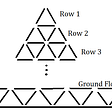# Limit divergence of polynomial fitting

I am approximating a set of data with a polynomial to later find the cumulative density function. I am using a polynomial since I do not know the distribution of the set of data, that may even vary, and because it should be fully automatized. The problem is thatat the end of my set of data, once the polynomial reaches the last data, divergest to infinity. Since it should approximate a probability density function it shouldn’t happen, because like so it influence all the results. How can I fix that?

`p=polyfit(X,N,21);      x=linspace(0,4000,1000);      y=polyval(p,x);`

NOTE:-

Matlabsolutions.com provide latest MatLab Homework Help,MatLab Assignment Help for students, engineers and researchers in Multiple Branches like ECE, EEE, CSE, Mechanical, Civil with 100% output.Matlab Code for B.E, B.Tech,M.E,M.Tech, Ph.D. Scholars with 100% privacy guaranteed. Get MATLAB projects with source code for your learning and research.

The simple answer for this is to ask a question. WHY IN THE NAME OF GOD AND LITTLE GREEN APPLES ARE YOU USING A POLYNOMIAL????? Ok, maybe I’m being too hard on you. Polyfit is there for you to use. Nothing in the documentation tells you it does silly things, or that this is the fundamental nature of polynomials. Somewhere teachers should teach you, right after they tell you that you CAN use a polynomial, that you probably, usually, should not do so. And then explain why not.

Seriously, DON’T USE A POLYNOMIAL. And NEVER use a high order polynomial. They go to hell faster than a low order one. A polynomial order of 21? WAY TOO HIGH. But then you should not use a polynomial at all here.

Polynomials do exactly what you saw. They go to infinity. Think about it. what other behavior can you possibly expect? Can a polynomial approximate a function that does non-trivial (non-constant) stuff within some region, then goes constant as x goes to plus or minus infinity? Think about it. A polynomial can never be a model for a process that behaves like a CDF. You have this really high order polynomial, with powers as high as 21. X is as large as 4000?

What is 4000²¹? How about other numbers near there?

`4000^21ans =    4.398e+753950^21ans =   3.3771e+753500^21ans =   2.6634e+74`

The highest power of X with a non-zero coefficient will dominate the behavior of a polynomial as X goes to +/-inf. So your high order polynomial produces complete and utter garbage out there. You know this is the behavior that you see. And simple mathematics tells you that you cannot expect any other behavior from a polyomial model. That is exactly the behavior you expect from a polynomial, the innate, fundamental behavior that exists in any polynomial, and something you cannot change.

The obvious answer to my original question is you have no idea what else you might use. Sorry, but that is clearly true.

And, the answer to that is to use a spline. There are several choices you might use there. The simplest is pchip, which will interpolate your data. (Of course, you could have just tried interp1, which can use pchip, or various options as you would direct it to use.)

--

--

--

## More from Technical Source

Simple! That is me, a simple person. I am passionate about knowledge and reading. That’s why I have decided to write and share a bit of my life and thoughts to.

Love podcasts or audiobooks? Learn on the go with our new app.

## Solution Insights: Problem on Geometry for 28–01–2022## The beauty of mathematics is not obvious…but we can make it so## On Estimation## Grover’s Algorithm: Quantum Algorithms Untangled## Logarithm and mathematical hack## The Most Common Positions for Chess Pieces## Squiggles on the blackboard## 4 Fun Math Tricks For Easy Calculation## Technical Source

Simple! That is me, a simple person. I am passionate about knowledge and reading. That’s why I have decided to write and share a bit of my life and thoughts to.

## How plot a matrix for Naive Bayes classifier?## PROFIT PREDICTION USING LINEAR REGRESSION## Implementing Gradient Descent in Python## 🍚 Basic Machine Learning Recipe